# Convert Month Name to Number

This post will guide you how to convert month name to number in excel. How do I convert month name to number with an excel formula. How to convert month name to number with an Excel VBA macro. How to convert 3 letter month abbreviation to number in excel. How to convert 3 character month to number in excel.

Assuming that you have a list of data B1:B12 contain month names and you want to convert all month names to numbers, how to achieve it.

## Convert Month Name to Number with Excel Formula

If you want to convert month name to number with an excel formula, or you want to convert 3 letter month name to numbers, you can create a formual based on the MONTH function and the DATEVALUE function. Like this:

`=MONTH(DATEVALUE(B1&"1"))`

Type this formula into the formula box of a blank cell C1, then drag the AutoFill handler over other cells to apply this formula.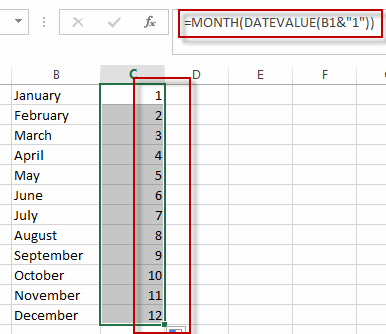You will see that all month names have been converted to numbers in range of cells C1:C12.

## Convert Month Name to Number with VBA code

You can also use an excel VBA Macro to convert month name to number in excel. Just do the following steps:

#1 open your excel workbook and then click on “Visual Basic” command under DEVELOPER Tab, or just press “ALT+F11” shortcut.#2 then the “Visual Basic Editor” window will appear.

#3 click “Insert” ->”Module” to create a new module#4 paste the below VBA code into the code window. Then clicking “Save” button.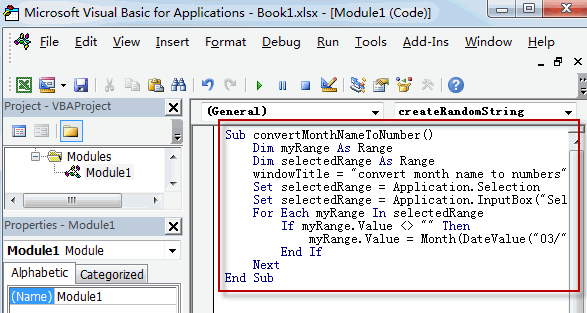```Sub convertMonthNameToNumber()
Dim myRange As Range
Dim selectedRange As Range
windowTitle = "convert month name to numbers"
Set selectedRange = Application.Selection
Set selectedRange = Application.InputBox("Select one Range that contain month names", windowTitle, selectedRange.Address, Type:=8)
For Each myRange In selectedRange
If myRange.Value <> "" Then
myRange.Value = Month(DateValue("03/" & myRange.Value & "/2018"))
End If
Next
End Sub```

#5 back to the current worksheet, then run the above excel macro. Click Run button.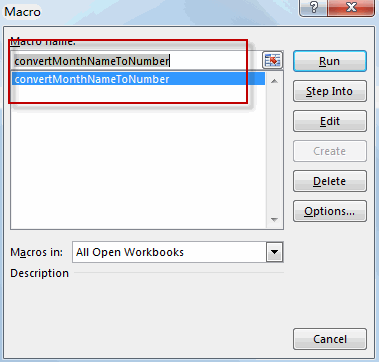#6 select cells that you want to convert, such as: B1:B12. Click Ok button.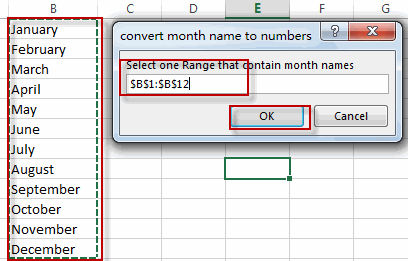#7 Let’s see the result.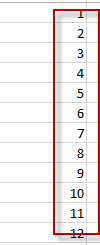## Convert Number to Month Name with Excel Formula

If you want to conert number to month name, you can use an formula based on the TEXT function, and the DATE function to achieve it. Like this:

`=TEXT(DATE(2018,B1,1),"mmmm")`

Type this formula into a blank cell, and then press enter key, then drag the AutoFill Handler over other cells.

Let’s see the result.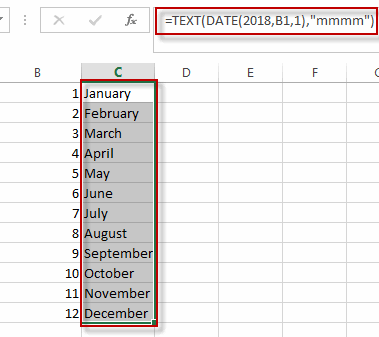### Related Functions

• Excel MONTH Function
The Excel MONTH function returns the month of a date represented by a serial number. And the month is an integer number from 1 to 12.The syntax of the MONTH function is as below:=MONTH (serial_number)…
• Excel DATEVALUE Function
The Excel DATEVALUE function returns the serial number of date. And it can be used to convert a date represented as text format into a serial number that recognizes as a date format.The syntax of the DATEVALUE function is as below:=DATEVALUE(date_text)…
• Excel DATE function
The Excel DATE function returns the serial number for a date.The syntax of the DATE function is as below:= DATE (year, month, day)…
• Excel Text function
The Excel TEXT function converts a numeric value into text string with a specified format. The TEXT function is a build-in function in Microsoft Excel and it is categorized as a Text Function. The syntax of the TEXT function is as below: = TEXT (value, Format code)…